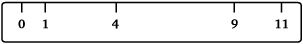This web site was created as supportive material for my diploma thesis "An analysis of the Golomb ruler and the Sidon set problems, and determination of large near-optimal Golomb rulers", submitted to the department of Electronic and Computer Engineering at Technical University of Crete (June 2002).   The discrete mathematics problems of Sidon sets and Golomb rulers  have been studied since the 1930's and 1960's respectively by non-overlaping groups of researchers. The main contribution of this diploma thesis was a study of the relationship between the two problems and the computational verification of a conjecture by Erdös on Sidon sets up to a much larger bound than the one previously known.   All the the source code used and the resulting Golomb rulers used for the proof of the main result of this thesis can be found at the bottom of this page. What are Golomb rulers and Sidon sets?   Golomb rulers are sets of positive integer numbers having all the differences between any pair of elements of the set to be unique. These numbers  can be thought of as ruler  marks (at integer locations) as an analogy with common rulers.  Golomb rulers have many applications ranging from constructions for error correcting codes, to placement of radio telescopes in linear arrays. They were first studied by Babock in 1953 who was led to their definition to solve a problem in inteference between communication  channels. Golomb was the first researcher to systematically study the subject in the 1960's  and since then his name is associated with these constructions.  The function G(n), referred to as the length of an optimal Golomb ruler , is defined as the smallest possible length of a ruler with n marks.   Sidon sets or B2 sequences is a related problem from combinatorial number theory. These sequences are subsets of 1,2,...,n having distinct pairwise sums between the elements. Sidon sets are named after Fourier analyst Simon Sidon who defined these sets in order to solve a problem in harmonic analysis. Sidon communicated the problem to Erdös who, together with Paul Turan, made the first publication on the topic in 1934. It was Erdös who gave the name Sidon sets to these constructions. The function F2(n) is defined as the largest number of element which can be selected from the first n positive integers forming a Sidon set. Diploma Thesis The full text of the thesis is available online in the following formats: Postscript file: main.ps.gz (1.1MB) PDF file: main.pdf (5.1MB) The slides of the original presentation are available (in Greek): PDF file: presentation.pdf (3.8MB) The presenation at University of Toronto (in English): PDF file: presentation.pdf (3.9MB) Source Code   Source code is i C++, and compiles well under GCC. However it uses some GCC extensions like long long for 64-bit integers and therefore it needs some modifications to compile under other compilers. README.txt Instructions for running the software. ruzsa.cpp Uses Ruzsa's construction to find near-optimal Golomb ruler lengths. ruzsa-reconstruct.cpp Uses parameters from the previous program to construct the actual rulers. bose-fast.cpp Use's Bose-Chowla construction and finds near-optimal Golomb ruler lengths. bose-reconstruct.cpp Reconstructs the rulers from the data of the previous program. common.cpp Common subroutines used by all the following programs. sort.cpp Sorting functions   Compile any of the above programs using g++, for example: g++ -O2 -o ruzsa ruzsa.C Results and tables of Golomb rulers up to 65000 marks Golomb rulers were found by exhaustive search of Ruzsa's construction for up to 65000 marks. The resulting Golomb ruler sizes are contained in the following file:  This text file contains 5 integer number in each line. The format of each line is: n   p   l   g   m where: n: number of marks of the Golomb ruler found p: prime number used for Ruzsa's construction l: length of the resulting Golomb ruler g: primitive element of Z_p used m: multiplier used  The actual Golomb rulers are not contained since it would require about 20GB of storage. The first 1000 Golomb rulers are contained in the following file:   For the remaining Golomb rulers use the ruzsa-reconstruct program with suitable parameters to find the actual rulers. Or type here the number of marks and the resulting Golomb ruler will be returned: number of marks:     Related Sites (for more information contact me at the email address shown at the top of this page) Back to my home page Dimitromanolakis Apostolos